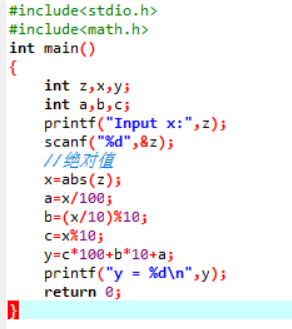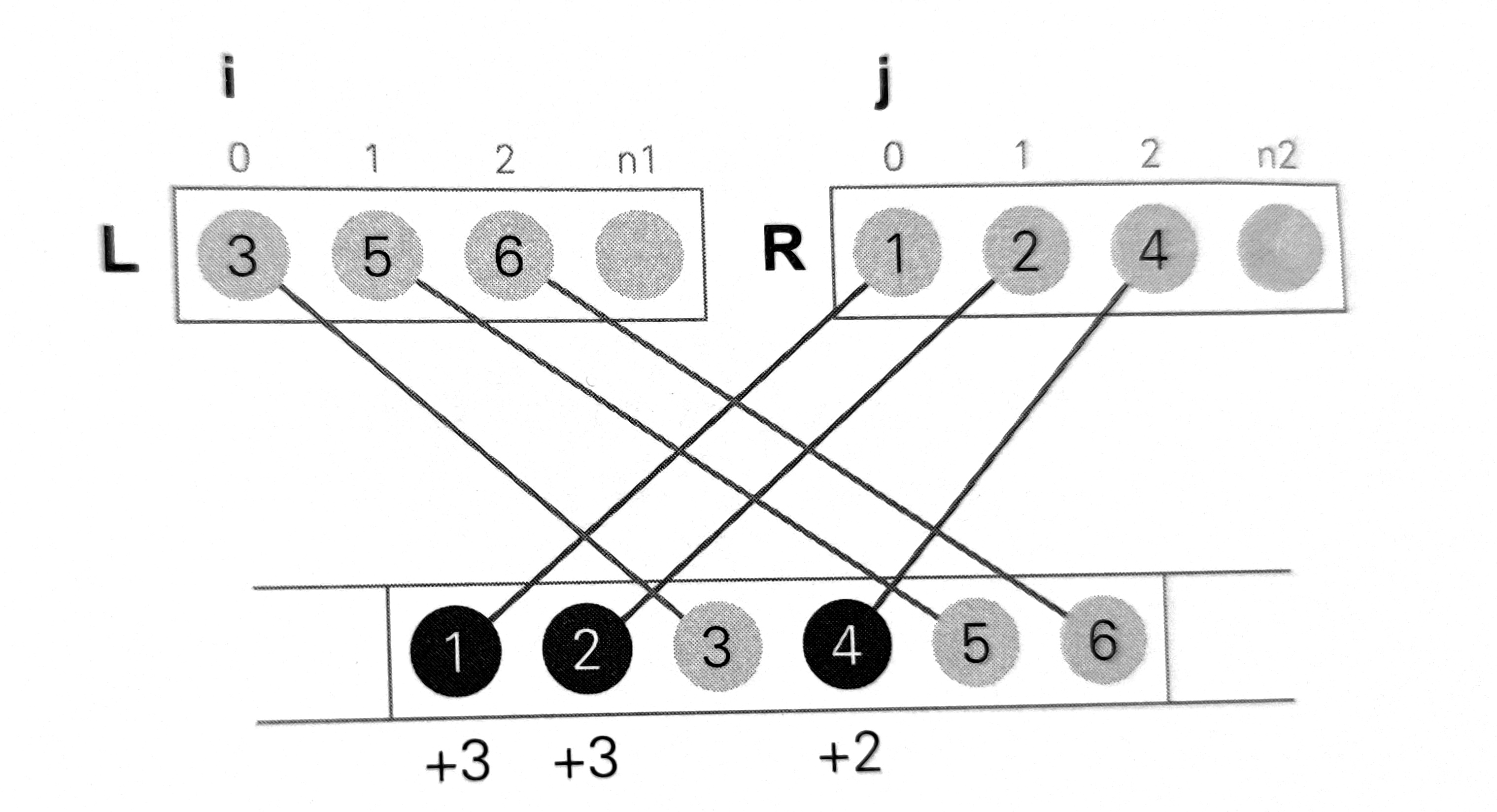• 4012: 计算逆序数
2019-07-07 15:31:11

4012: 计算逆序数
题目描述
给定n个互不相同的整数的序列a1, …, an。如果i<j且ai>aj，则称(ai,aj)是一个逆序。例如，5个数的序列
2, 4, 1, 3, 5
该序列中有3个逆序：(2, 1)、(4, 1)和(4, 3)。
设计一个分治算法计算一个序列中的逆序数。

输入
输入包括多组数据。每组数据有两行。每组数据的第一行是一个整数n(100 <= n <= 1000)，n = 0意味着输入结束；第二行包含构成序列的n个整数。

输出
对每组测试数据，输出序列中的逆序数。

样例输入

5
2  4  1  3  5
7
2  7  1  4  5  3  9
0


样例输出

3
7


答案如下

#include<stdio.h>
int main()
{
int a,i,j,k,n,t=0;
for(k=0;;k++)
{
scanf("%d",&n);
if(n==0)
break;
for(i=0;i<n;i++)
scanf("%d",&a[i]);
for(i=0;i<n;i++)
{
for(j=i+1;j<n;j++)
{
if(a[j]<a[i])
t++;
}
}
printf("%d\n",t);
t=0;
}
return 0;
}

更多相关内容
• ## C语言计算逆序数

千次阅读 2022-03-28 16:20:32
从键盘任意输入一个3为整数，编程计算并输出它的逆序数（忽略整数前的正负号）。例如，输入-123，则忽略负号，由其百位1、十位2、个位3，然后计算3*100+2*10+1 = 321，并输出321。 输入格式要求："%d" 提示信息：...

从键盘任意输入一个3为整数，编程计算并输出它的逆序数（忽略整数前的正负号）。例如，输入-123，则忽略负号，由其百位1、十位2、个位3，然后计算3*100+2*10+1 = 321，并输出321。

输入格式要求："%d" 提示信息："Input x:"

输出格式要求："y = %d\n"

程序运行示例如下：

Input x：-123

y = 321展开全文c++
• 计算逆序数 在很早之前，我曾经发过一篇文章，讲的是冒泡排序的交换次数就是逆序数。可是，这样计算逆序数的话，时间成本就很高，比较冒泡是时间复杂度为O(N²)的算法呢！那怎么办呢？其实，我们可以使用归并排序的...

## 计算逆序数

在很早之前，我曾经发过一篇文章，讲的是冒泡排序的交换次数就是逆序数。可是，这样计算逆序数的话，时间成本就很高，比较冒泡是时间复杂度为O(N²)的算法呢！那怎么办呢？其实，我们可以使用归并排序的思想来计算逆序数。

（以下内容需要先了解归并排序，具体讲解可以看我的这一篇文章：）

【笔记】数据结构之归并排序

我们会发现，在进行升序的归并排序时，每一次后方元素移到前面来的移动距离就是本次操作的逆序数。那么我们思考之后可以得出，每一步操作的逆序数就是n1-i

具体得看下面这个图：由于每一层递归结束之后，左右两边都变成了已经升序排序的数组，那么自然地，当右边的元素小于左边元素的时候，把它移到前面的逆序数就是n1-i

## 代码实现

#include<iostream>
using namespace std;

unsigned int L,R;
unsigned int num;
unsigned long long nixushu=0;

void _Merge(int left, int mid, int right)
{
int n1 = mid-left;
int n2 = right-mid;

for(int i=0;i<n1;i++)
L[i] = num[left+i];

for(int i=0;i<n2;i++)
R[i] = num[mid+i];

L[n1]=R[n2]=1000000003;
int js1=0,js2=0;

for(int i=left;i<right;i++)
{
if(L[js1]<=R[js2])
{
num[i] = L[js1];
js1++;
}
else
{
num[i] = R[js2];
js2++;
nixushu += n1-js1;

}
}

}

void mergeSort(int left, int right)
{
if(left+1>=right)
return;

int mid = (left+right)/2;
mergeSort(left,mid);
mergeSort(mid,right);
_Merge(left, mid, right);

}

int main()
{
int n;
cin>>n;
for(int i=0;i<n;i++)
cin>>num[i];
mergeSort(0,n);
cout<<nixushu<<endl;
}


因此，我们需要关注的关键问题就是合并函数的实现。经过上面的分析，我们可以知道，我们只需要在归并排序的合并函数里面，负责处理L[js1]>R[js2]的那部分代码里面做一些修改，就可以实现计算逆序数的目的。欢迎关注我的公众号哦！

展开全文算法 数据结构 排序算法
• 本文简单介绍了几种计算逆序数的实现方法 简介 所谓逆序数,是指一个排列中所有逆序对的总数,而所谓逆序对,则是指排列中前后位置和大小顺序相反的数对,举个简单的例子: { 1,4,2,3 } \{\ 1, 4, 2, 3 \ \} {...

本文简单介绍了几种计算逆序数的实现方法

#### 简介

所谓逆序数,是指一个排列中所有逆序对的总数,而所谓逆序对,则是指排列中前后位置和大小顺序相反的数对,举个简单的例子:

{   1 , 4 , 2 , 3   } \{\ 1, 4, 2, 3 \ \}

上面的排列中,有下面两个逆序对:

< 4 , 2 >   < 4 , 3 > <4, 2>\ <4, 3>

所以该排列的逆序数为 2

#### 实现

• 朴素的实现很简明,我们遍历排列中所有的数对,检查是否形成逆序关系即可,示例代码如下(Lua):
function inverse_number(seq)
local inv_num = 0

for i = 1, #seq - 1 do
for j = i + 1, #seq do
if seq[i] > seq[j] then
inv_num = inv_num + 1
end
end
end

return inv_num
end

• 上面的方法虽然简明,但是时间复杂度相对较高( O ( n 2 ) O(n^2) ),这里我们假设排列中元素种类很少(譬如只有 1 , 2 , 3 , 4 1, 2, 3, 4 ),那么更有效率的一种实现方法就是依次遍历排列元素,对于每一个遍历到的元素而言,该元素之前所有比他(该元素)大的元素,与该元素便形成了一个逆序对(即逆序数增一),依此我们便可以累加计算出排列的逆序数(Lua):
-- assume seq contains "1, 2, 3, 4" only
function inverse_number(seq)
local inv_num = 0

local count_buffer = { 0, 0, 0, 0 }

for i = 1, #seq do
if seq[i] == 1 then
inv_num = inv_num + count_buffer + count_buffer + count_buffer
count_buffer = count_buffer + 1
elseif seq[i] == 2 then
inv_num = inv_num + count_buffer + count_buffer
count_buffer = count_buffer + 1
elseif seq[i] == 3 then
inv_num = inv_num + count_buffer
count_buffer = count_buffer + 1
else
count_buffer = count_buffer + 1
end
end

return inv_num
end

• 上面代码的时间复杂度虽然比较高效( O ( n ) O(n) ),但是通用性不高(限制了排列元素种类),我们可以简单扩展一下(Lua):
function inverse_number(seq)
local inv_num = 0

local count_buffer = {}

for i = 1, #seq do
for k, v in pairs(count_buffer) do
if k > seq[i] then
inv_num = inv_num + v
end
end

count_buffer[seq[i]] = (count_buffer[seq[i]] or 0) + 1
end

return inv_num
end

• 实际上而言,上面实现的时间复杂度也是 O ( n 2 ) O(n^2) ,但在元素种类受限的排列中,使用该实现来求取逆序数的速度仍是非常快的,另外的,我们还可以借用树状数组来进一步加速,有兴趣的朋友可以继续看看更多资料里的内容.

#### 更多资料

展开全文数学
• 有一实数序列a1,a2,....an，若i且ai>aj，则（ai，aj）形成了一个逆序对，请使用分治算法求整个序列中逆序对个，并分析算法时间复杂度。
• 文章目录逆序数对简介计算逆序数对思路significant inversion 简介significant inversion 思路significant inversion 代码 逆序数对简介 数组中的两个元素A[i], A[j]，如果下标 i < j，但 A[i] > A[j] ，称 A...算法
• 学到行列式的时候，每次遇到对给定的序列计算逆序数，她都觉得是个很闹心的事。所以，她央求她的好朋友 Ray 为她写一段程序，用来解决这样的问题。作为回报，她答应在周末舞会上让 Ray 成为她的伦巴舞舞伴。所谓...c++
• } 那么有一个整数，我们不知道它是几位数，那么应该怎样实现他的逆序数呢？ #include int main() { int x, i,sum = 0; int n = 0;//表示是n位数。 int a,b,y; scanf("%d", &x); y = x; while(y != 0) { y = y/10; n...c语言
• ## 计算逆序对/逆序数

千次阅读 2016-04-15 23:50:37
利用归并排序计算逆序对 #include &lt;iostream&gt; #include &lt;cstring&gt; #include &lt;cstdio&gt; #include &lt;cmath&gt; #include &lt;algorithm&gt; #include &...归并排序
• 计算一个tuple里面的逆序数，用merge sort的办法。我写了以下代码，但是每次统计的时候，count设置为全局变量了：'''Count inversionInput: a sequence as tuple of integersOutput: The inversion number as an ...
• 线段树计算逆序数的原理： 用线段树来统计已插入的数的个数（所以要保证最大的那个数不能太大，否则数组都开不了），然后每插入一个数，就查询比插入的数大的个数，累加即可。 这个题还有一个特点就是，题目给的是...
• 归并排序是将数列a[l,h]分成两半a[l,mid]和a[mid+1,h]分别进行归并排序，然后再将这两半合并起来。...a[j]时，在前半部分中比a[i]大的数都比a[j]大，将a[j]放在a[i]前面的话，逆序数要加上mid+1-i。因此...
• #include #include #include int Divid(int* a, int* b, int first, int last) {  if(first >= last) return 0; // 一个元素的情况下没有逆序数  int i,j,k=0,mid;  k=i=first;  int res
• ## 分治法求逆序数

千次阅读 2019-03-15 11:14:22
// 计算逆序对数  count = count + len1 - i + 1;  // 排序 生成新的有序数组  Arr2[p + k] = arrR[j];  j++;  }  else  {  Arr2[p + k] = arrL[i];  i++;  }  }  return count; } ...分治法 归并排序
• /*----------------------... 名称：Use divide-and-couquer count the inversion 分治法计算逆序数 编写：MISaD 博客：http://blog.csdn.net/misadd 日期：2014.10.25 修改：2014.10.25 主要错误在于1.错误估
• 最近在刷算法导论，在第二章思考题2-4的d问题，提示使用递归排序计算序列的逆序数。基本思想如下，归并排序的内容见我上一篇文章https://blog.csdn.net/weixin_44004576/article/details/102635900 递归每次将序列...算法导论 归并排序 C++
• 一个排列中逆序的总数就称为这个排列的逆序数。一个排列中所有逆序总数叫做这个排列的逆序数。也就是说，对于n个不同的元素，先规定各元素之间有一个标准次序（例如n个 不同的自然数，可规定从小到大为标准次序），...
• 在数组中的两个数字，如果前面一个数字大于后面的数字，则这两个数字组成一个逆序对。输入一个数组,求出这个数组中的逆序对的总数P。并将P对1000000007取模的结果输出。 即输出P%1000000007 public class test { ...
• //求整数n的逆序数并返回：如1234的逆序数为4321；4的逆序数为4 int Reverse(int n){ int rev = 0;//rev作为最终返回结果，通过不断对n进行取余和除法使rev逐渐形成 while(n>0){ rev = rev*10 + n%10; n = n/...c++
• 求输入数据后求逆序数问题。常用于本科，研究生的算法作业。里面是工程文件，可以直接使用。
• ## Python求取逆序数

千次阅读 多人点赞 2021-03-18 10:09:29
Python求取逆序数方法一.py方法二.py方法三.py 方法一.py #利用简单的数学计算 num = int(input('请输入一个三位正整数：')) a = num//100 b = num%100//10 c = num%100%10 print('该数的反序数为：',(100*c+10*b+a)...python 算法
• 即在一个排列中，我们计算每个数字后面，比它本身小的数字的个数，最后将个数相加即为列表的逆序数。 ans = 0 a = [1,2,6,3,5,4] for i in range(len(a)):# 循环列表 for j in range(i):# 判断该数字后是否有比它...python 算法
• ## matlab求逆序数

千次阅读 2018-07-18 00:31:27
matlab求逆序数 逆序数概念： 因为没时间详细介绍逆序数概念，上传图片仅作参考。 逆序数matlab代码： clc clear %author:猪猪侠 %date:2018-7-18 x=input('请输入数据');%输入数据 str=num2str(x);%转换...
• 解题思路:归并排序 只不过加了个记录变量ans注意事项:参考代码:#includelong long ans = 0;int a, b, n;void merge(int low, int mid, int high) {int i, j, k;for (i = low, j = mid + 1, k = i;...
• ## 逆序数的几种求法

万次阅读 2018-08-03 14:01:30
首先，逆序数的定义 什么叫逆序数 对于某一个数来说，它的逆序数等于在它之前有多少个比它大的...对于某一个序列来说，逆序数等于所有数的逆序数之和 例如  序列 5 1 5 2 逆序数 0 1 0 2 序列的逆序数 1+2...
• 逆序数即为将数字位数反过来的数，比如说12345的逆序数是54321 题目描述 现在给你一个数x，请你输出它的逆序数 输入格式 输入共一行，一个整数x 输出格式 输出共一行，一个整数y表示x的逆序数 输入输出样例 输入 #1 ...
• ## 求逆序数（C语言）

千次阅读 2020-03-26 21:26:03
int reverse(int s){ int sum=0; while(s!=0){ sum*=10; sum+=(s%10); s/=10; } return sum; } int main(){ int s,x; scanf("%d",&s); while(s!=-1){ printf("%d\r\n",reverse(s))... scanf("%...
• j，那么这组数就称为一个逆序，数列A中逆序的数量称为逆序数逆序数与下述冒泡算法的交换次数相等。 bubbleSort(A) cnt = 0 //逆序数 for i = 0 to A.length - 1 for j = A.length - 1 downto i + ...算法 分治法 C++
• 在一个序列中，例如{ 2, 4, 3, 1 } ，逆序依次为 (2,1), (4,3), (4,1), (3,1)，因此该序列的逆序数为 4。 【输入形式】 输入包括两行，第1行n表示序列元素的个数，第2行n个正整数，表示求逆序数的序列。 【输出形式...C++ 程序设计...